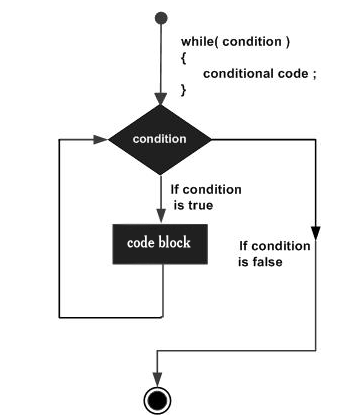×

Search anything:

# While loop control statement

#### control statement while loop loopGet this book -> Problems on Array: For Interviews and Competitive Programming

A while loop is a control flow statement that allows code to be executed repeatedly based on a given condition.

Syntax

``````
while(condition)
{
statement(s);
}
``````

Here, statement(s) may be a single statement or a block of statements. The condition can be a logical or arithmatical combination of multiple small expressions which evaluate to true or any non-zero value. The loop iterates while the condition is true.

When the condition becomes false, program control passes to the instruction immediately after the loop.Note:-while loop might run forever which, will result as error in our source program.

When the condition is evaluated and the result is true, loop will be executed. We need to take care that after finite number of time our condition result into false in order to terminate execution.

## Example

Program to print all natural number less than or equal to '6' using While loop.

``````
int main()
{
int i=1;
/* The loop would continue to print
* the value of i until the given condition
* i<=6 returns false.
*/
while(i<=6){
cout<<"Value of variable i is: "<<i<<endl; i++;
}
}
``````

When the above code is compiled and executed, it produces the following result :-

``````
Output

Value of variable i is: 1
Value of variable i is: 2
Value of variable i is: 3
Value of variable i is: 4
Value of variable i is: 5
Value of variable i is: 6
``````#### Tanya Anand

Final Year Undergrad (2020) | CSE | NIT Hamirpur | Intern at OpenGenus

While loop control statement